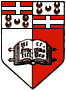CHE1200 - Mathematics for Chemists Dr. Joseph N. Grima Department of Chemistry University of Malta, Msida, MSD 06, MALTA

Tutorial Sheets:

 01 | 02 | 03 | 04 | 05 | 06 | RESOURCES

 Credit Value: 4 ECTS No. of Lectures: 28 No. of Tutorials/Labs: 28 Year: 1 Semester: 1 & 2 Pre-requisite: O-Level Maths
Syllabus:
[TOP]
2. Calculus (Theory):
• Differentiation: Differentiation of basic functions, product rule, quotient rule, minima and maxima, function of a function, chain rule, curve sketching and essential coordinate geometry, complex numbers, Maclaurin and Taylor Series.
• Integration: Integration of basic functions. Integration by substitution, integration by parts, finite integration, numerical integration.
• Functions of several variables and partial differentiation.
• Differential equations: First and second order differential equation, boundary

• conditions.
[TOP]
3. Calculus (Applications):
• Application of differentiation to locate and identify turning points.
• The role of calculus in thermodynamics.
1. The use of integration to calculate p-V work (i.e. to find the area under p-V graphs).
2. Integration as a means to obtain a 'measurable' change in a quantity, Dx, from infinitesimally small changes, dx.
3. Partial differentiation and state functions.
4. The use of partial derivatives to differentiate expressions of the sort G = H- TS, and then use these to find how, for example, G varies with T at constant p.
5. The role of differential equations in:
• Chemical kinetics;
• Quantum mechanics.
[TOP]
4. Matrices and determinants:
• Matrix notation
• Elementary matrix operations and properties
• Determinants
• Inverse matrices
• Eigenvalues and eigenvectors
[TOP]
5. Probability and statistics:
• Permutations and combinations
• Introduction to statistics
• Regression analysis
• Applications
6. Mathematics through computers:
• Plotting of curves, data analysis, etc.
[TOP]

Method of assessment:

Course work 25%
End of year test